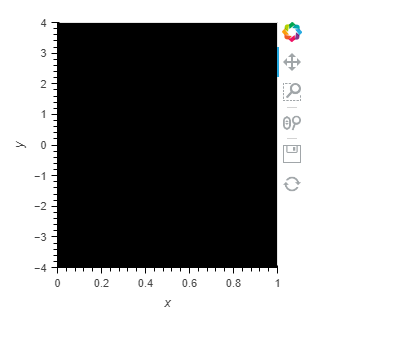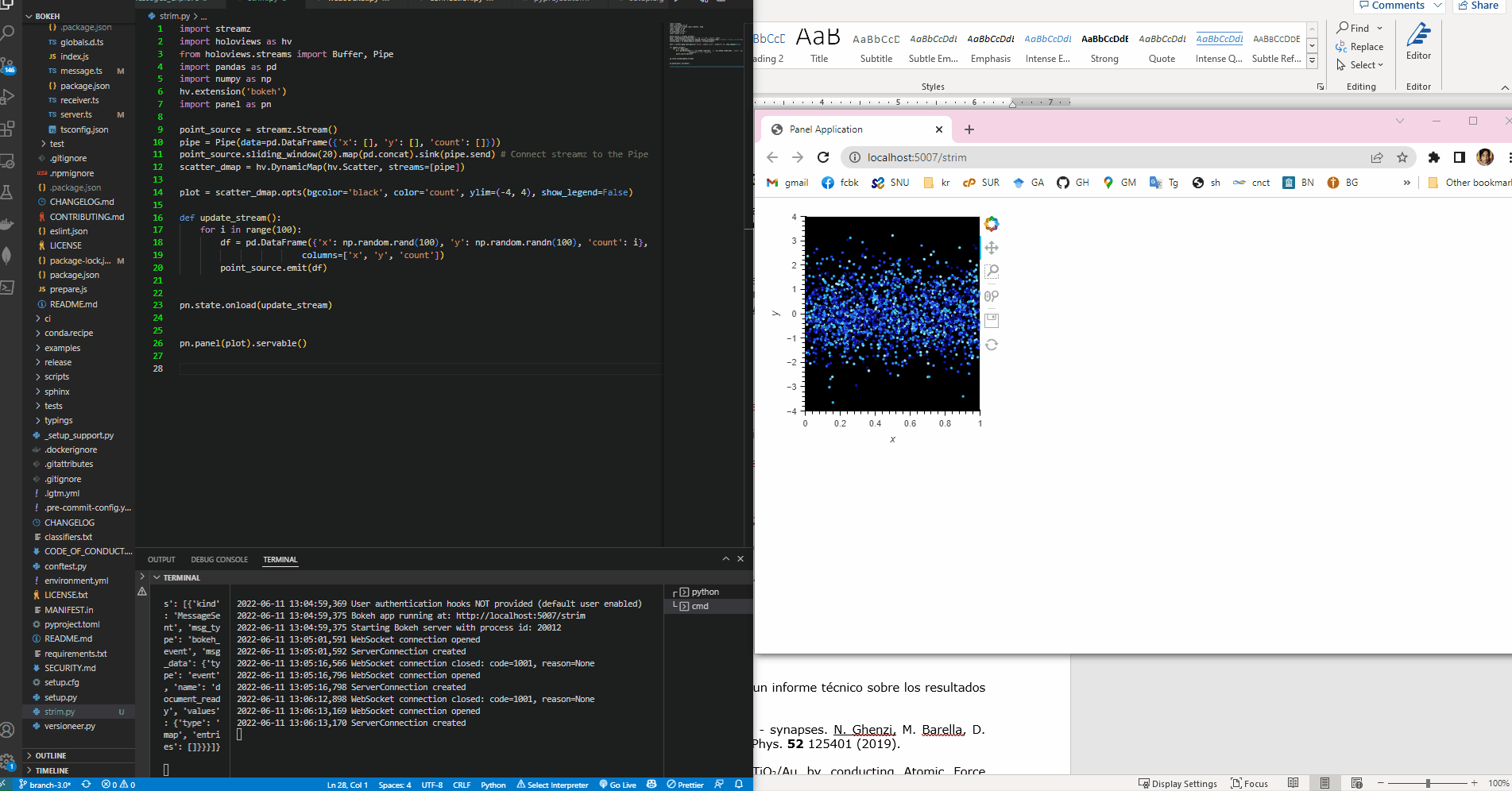# Plotting streamz + pipe example in a browser not working

Hi, I’m trying to plot a dynamic map from PyCharm and render it in a new browser window. I’m trying to get this working. Here’s my code:

``````import streamz
import holoviews as hv
from holoviews.streams import Buffer, Pipe
import pandas as pd
import numpy as np
hv.extension('bokeh')
import panel as pn

point_source = streamz.Stream()
pipe = Pipe(data=pd.DataFrame({'x': [], 'y': [], 'count': []}))
point_source.sliding_window(20).map(pd.concat).sink(pipe.send) # Connect streamz to the Pipe
scatter_dmap = hv.DynamicMap(hv.Scatter, streams=[pipe])

plot = scatter_dmap.opts(bgcolor='black', color='count', ylim=(-4, 4), show_legend=False)
pn.panel(plot).show()

for i in range(100):
df = pd.DataFrame({'x': np.random.rand(100), 'y': np.random.randn(100), 'count': i},
columns=['x', 'y', 'count'])
point_source.emit(df)

``````

But all I see in my browser is a black slate:From what I’ve understood, the panel() call should start a bokeh server that allows me to view streaming data. Have I understood wrong?

Also, for various reasons I can’t use a notebook, have to stick with PyCharm.
Thanks!

`pn.panel(plot).show() ` is for the notebook. For the server you should use

`pn.panel(plot).servable()`

with
panel serve --show filename.py

Thank you, but if I do that it the HTML page only shows up after the loop has finished. I only see the end result, I don’t see the data being streamed.

Also, I’m trying to run the first streaming example using Pipes in a notebook as follows:

``````import time
import numpy as np
import pandas as pd
import holoviews as hv
import streamz
import streamz.dataframe
from bokeh.plotting import show

from holoviews import opts
from holoviews.streams import Pipe, Buffer

hv.extension('bokeh')

pipe = Pipe(data=[])
vector_dmap = hv.DynamicMap(hv.VectorField, streams=[pipe])
vector_dmap.opts(color='Magnitude', xlim=(-1, 1), ylim=(-1, 1))

vector_dmap
x,y  = np.mgrid[-10:11,-10:11] * 0.1
sine_rings  = np.sin(x**2+y**2)*np.pi+np.pi
exp_falloff = 1/np.exp((x**2+y**2)/8)

for i in np.linspace(0, 1, 25):
time.sleep(0.1)
pipe.send((x,y,sine_rings*i, exp_falloff))
``````

But I only get a blank output cell. I’m definitely missing something basic, could you please let me know what it is? I assume the line `vector_dmap` should display the plot, but that’s not happening.

In the first approach if you add the .servable and wrap the streaming of the df with the pn.state.onload it works.``````import streamz
import holoviews as hv
from holoviews.streams import Buffer, Pipe
import pandas as pd
import numpy as np
hv.extension('bokeh')
import panel as pn

point_source = streamz.Stream()
pipe = Pipe(data=pd.DataFrame({'x': [], 'y': [], 'count': []}))
point_source.sliding_window(20).map(pd.concat).sink(pipe.send) # Connect streamz to the Pipe
scatter_dmap = hv.DynamicMap(hv.Scatter, streams=[pipe])

plot = scatter_dmap.opts(bgcolor='black', color='count', ylim=(-4, 4), show_legend=False)

def update_stream():
for i in range(100):
df = pd.DataFrame({'x': np.random.rand(100), 'y': np.random.randn(100), 'count': i},
columns=['x', 'y', 'count'])
point_source.emit(df)